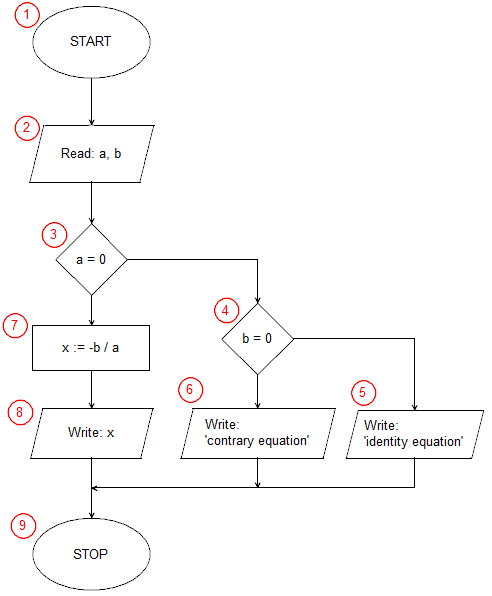# Implementation of the algorithm to solve linear equation in Pascal```program linear_equation;
var a, b, x: real;
begin
write('a = ');
write('b = ');
if a=0 then
if b=0 then
writeln('identity equation')
else
writeln('contrary equation')
else begin
x:=-b/a;
writeln('x = ',x);
end;
end.```

The first line of the program can be treated as an ornament, but required by the syntax of the language pascal. Every program in the pascal must start with the keyword program preceding the name of the program, it adds nothing to the implementation of the algorithm. The second line is auxiliary. We know that our algorithm uses three variables that store real numbers. In the pass, all variables must be declared before the block of code starts. When declaring a variable, we must specify what type of data will be stored in it.

The third line containing the key word begin corresponds to the starting block (1) of our algorithm.

Lines from 4 to 7 perform the input/output (2) operation in which the input data is taken - coefficients a and b of the equation.

The conditional statement from line 8 is the selection block (3) from our algorithm. The instruction checks if the coefficient a is equal to 0. When the condition is satisfied, the instruction after the then keyword is executed, written in lines 9 to 12 (in the algorithm block selection (4) and input-output blocks (5) and (6)). When the condition is not satisfied, the statement after the else keyword is executed, in our case it is a compound statement, enclosed in begin end logical brackets between lines 13 and 16. First, in line 14 we calculate the solution of the equation x = -b / a (block operational (7)), then on line 15 we print the result obtained (input-output block (8)).

The readln statement on line 17 has nothing to do with the execution of the our algorithm. It serves to stop the program until you press the enter key, which prevents the program window from closing immediately.

Instructions I. from line 18, the program ends (end block of the algorithm (9)).